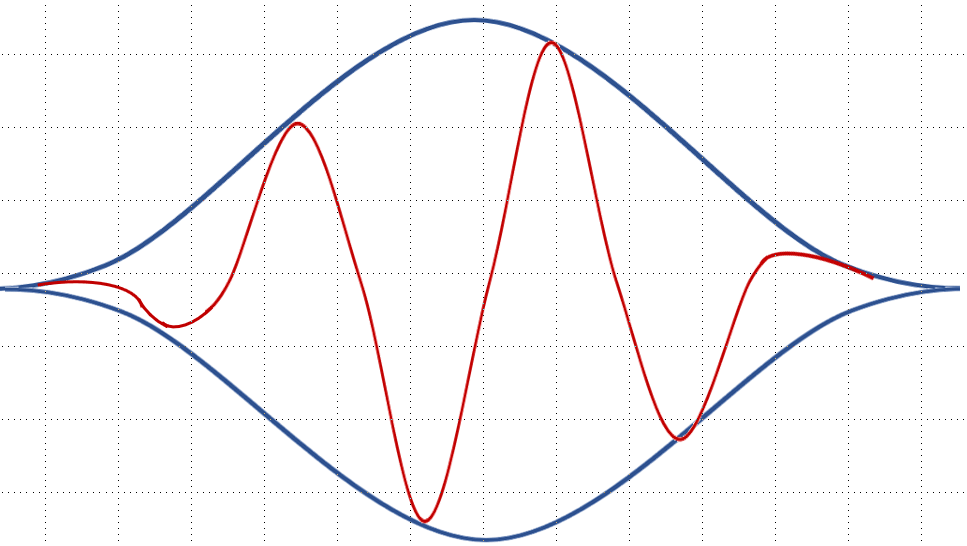# Why and How Window Functions Are Used

November 8, 2018

Fast Fourier transform (FFT) calculations are essential to signal processing. A signal processing algorithm moves data from the time domain into the frequency domain by performing a series of FFT calculations on time periods of equal size called frames.

However, the mathematics of the FFT assumes signals have an infinite, circular topology. The two endpoints of the time waveform are interpreted as though they were connected—i.e. have the same value at each end of a time interval. This is not true for a real-world random signal, and the resulting discontinuities in the time domain create unwanted noise in the frequency spectrum known as spectral leakage.Figure 1.1. Applying a windowing function.

### Discontinuities in the Time Domain

Window functions are added to a signal processing algorithm to address the discontinuity problem but they do not totally overcome it. These functions operate by multiplying the time waveform by a finite-length window with an amplitude that varies smoothly and gradually toward zero at the edges. However, multiplication in time does result in a distortion in frequency. So, while the FFT of the windowed waveform will be similar to the FFT of the original, it will have been altered.

#### Heisenberg Uncertainty Principle

Generally speaking, for a given window function, the larger the window in time (the more FFT analysis lines), the less distortion in frequency. The Heisenberg uncertainty principle summarizes this idea. Increasing the resolution in time (using a smaller window to zoom in on an interval of interest) results in a decreased resolution in frequency (more distortion). Conversely, decreasing resolution in time (using a larger window) results in an increased resolution in frequency (less distortion).

For a given window size (or number of analysis lines), different window functions will result in different distortion characteristics. All window functions are defined in both time and frequency. The exact characteristics of the resulting distortion are dependent on the definition of the selected window function.

Mathematicians and data scientists have developed a range of window functions. The following lessons explore the characteristics that describe these various functions and how they differ from the others.